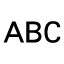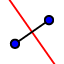# Circumcenter

A circumcenter is the point of concurrency of the three perpendicular bisectors. -Construct triangle XYZ and label the vertices with text tool. -Construct the perpendicular bisectorof each side. -Use the intersectin the point menu to mark the circumcenter and name it A with text tool.
Construct the circumcenter below.

Drag the vertices of XYZ around. What kind of triangle is XYZ if the circumcenter A falls on the exterior of the triangle?

Check all that apply

What kind of triangle is XYZ if the circumcenter A falls on the triangle?

Check all that apply

What kind of triangle is XYZ if the circumcenter A is in the interior of the triangle?

Check all that apply
A circumscribed circle (circle that goes through each vertex) can be added in this construction above. Draw a segment from the circumcenter A to vertex X. This creates the radius of the circle (segment AX). Construct a circle on your construction above using your compass tool. Move the triangle around and verify the circle always goes through all vertices X, Y and Z.

Drag around the vertices of XYZ. Does the circle always go through the three vertices and remain outside the triangle?

Check all that apply

Since a circumscribed circle goes through each vertex, the circumcenter is equidistant from each:

Check all that apply